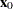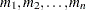RIDGE Statement
RIDGE <options> ;

A RIDGE statement computes the ridge of optimum response. The ridge starts at a given point, and the point on the ridge at radiusfromis the collection of factor settings that optimizes the predicted response at this radius. You can think of the ridge as climbing or falling as fast as possible on the surface of predicted response. Thus, the ridge analysis can be used as a tool to help interpret an existing response surface or to indicate the direction in which further experimentation should be performed.

The default starting point,, has each coordinate equal to the point midway between the highest and lowest values of the factor in the design. The default radii at which the ridge is computed are 0, 0.1,, 0.9, 1. If the ridge analysis is based on the response surface fit to coded values for the factor variables (see the section Coding the Factor Variables for details), then this results in a ridge that starts at the point with a coded zero value for each coordinate and extends toward, but not beyond, the edge of the range of experimentation. Alternatively, both the center point of the ridge and the radii at which it is to be computed can be specified.

You can specify the following options in the RIDGE statement:

CENTER=uncoded-factor-values

gives the coordinates of the pointfrom which to begin the ridge. The coordinates should be given in the original (uncoded) factor variable values and should be separated by commas. There must be as many coordinates specified as there are factors in the model, and the order of the coordinates must be the same as that used in the MODEL statement. This starting point should be well inside the range of experimentation. The default sets each coordinate equal to the value midway between the highest and lowest values for the associated factor.

MAXIMUM
MAX

computes the ridge of maximum response. Both the MIN and MAX options can be specified; at least one must be specified.

MINIMUM
MIN

computes the ridge of minimum response. Both the MIN and MAX options can be specified; at least one must be specified.

NOPRINT

suppresses the display of the ridge analysis when only an output data set is required.

OUTR=SAS-data-set

creates an output SAS data set containing the computed optimum ridge.

For details, see the section OUTR=SAS-data-set.

gives the distances from the ridge starting point at which to compute the optima. The values in the list represent distances between coded points. The list can take any of the following forms or can be composed of mixtures of them:specifies several values.TOspecifies a sequence whereequals the starting value,equals the ending value, and the increment equals 1.TOBYspecifies a sequence whereequals the starting value,equals the ending value, andequals the increment.

Mixtures of the preceding forms should be separated by commas. The default list runs from 0 to 1 by increments of 0.1. The following are examples of valid lists.

```radius=0 to 5 by .5;
radius=0, .2, .25, .3, .5 to 1.0 by .1;
```